<

# Perl 6 and the Real World - Structure

• What is a model? When is it a good model?
• A simple model
• Math: derivatives
• Free fall, spring
• Resonance

# What is a Model?

• physics = striving to understand (parts of) the world
• the world is too complicated
• models are descriptions that focus on one aspect
• so Model = Simplification

# Example Model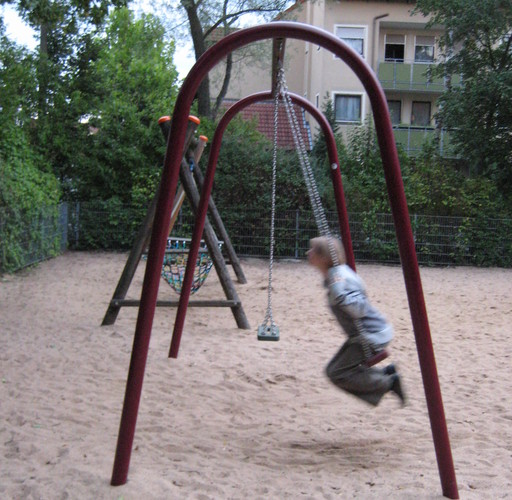# Example Model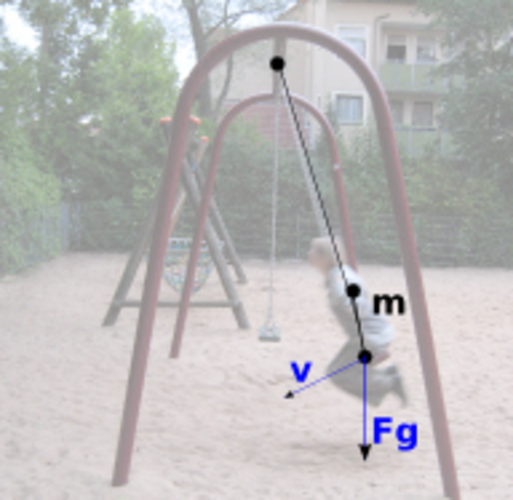# Model takes into account

• gravity
• inertia
• initial motion
• connection to anchor point

# Model neglects

• colors
• exact shapes
• size of object
• friction

# Is it a good model?

• it's a good model if it can answer a question for us
• examples "how fast is the object?", "What is the swinging period?", "Does the distance to the anchor point matter?"
• accuracy of the answer important
• every model needs input data. Is that available?
• extensibilty

# Another model: free falling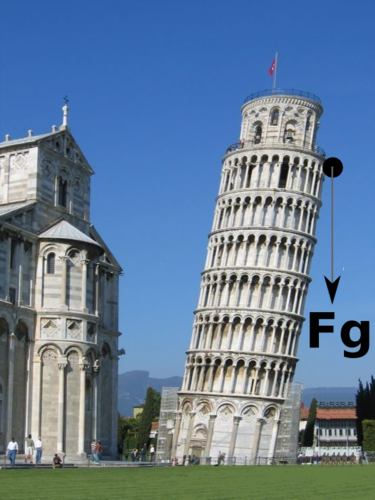# Free falling: Solved in Perl 6

```use Math::Model;

my \$m = Math::Model.new(
derivatives => {
velocity      => 'height',
acceleration  => 'velocity',
},
variables   => {
acceleration  => { \$:gravity },   # m / s**2
gravity       => { -9.81 },       # m / s**2
},
# ...
```

# Free falling: Solved in Perl 6

```    # ...
initials    => {
height        => 50,              # m
velocity      => 0,               # m/s
},
captures    => ('height', 'velocity'),
);

\$m.integrate(:from(0), :to(4.2), :min-resolution(0.2));
\$m.render-svg('free-fall.svg', :title('Free falling'));
```

# Model result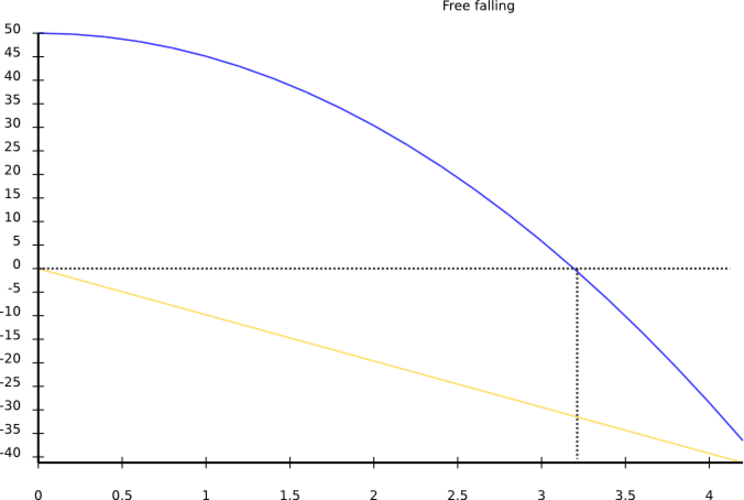# The model in detail

```use Math::Model;

my \$m = Math::Model.new(
derivatives => {
velocity      => 'height',
acceleration  => 'velocity',
},
```

# Derivative: slope of another quantity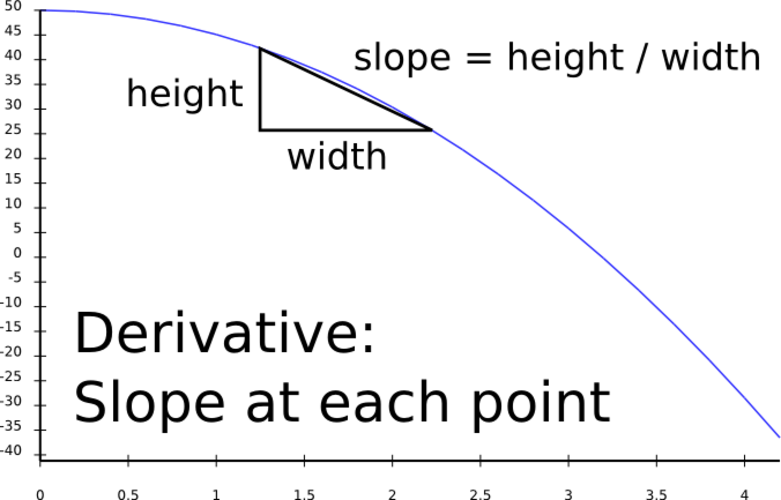# Common derivatives in Mechanics

```Derivative          Of

velocity            position
angular velocity    angle
acceleration        velocity
power               energy
force               momentum
(= mass * velocity)
```

# Common derivatives

```current             charge

birth rate          population
- mortality rate

profit              funds
```

# Using derivatives

• with Math::Model, you only need to know the derivatives, note the values derived from
• you need an initial value for the derived quantity
• (Ordinary Differential Equation, which Math::Model integrates for you)

# Rest of the model

```    variables   => {
acceleration  => { \$:gravity },   # m / s**2
gravity       => { -9.81 },       # m / s**2
},
initials    => {
height        => 50,              # m
velocity      => 0,               # m/s
},
captures    => ('height', 'velocity'),
);

\$m.integrate(:from(0), :to(4.2), :min-resolution(0.2));
\$m.render-svg('free-fall.svg', :title('Free falling'));
```

# Perl 6 stuff

• `\$:height` is a named parameter
• `Math::Model` introspects code blocks for arguments
• calculates dependencies => execution order
• RungeKutta integration

# Extending the model - Spring, damping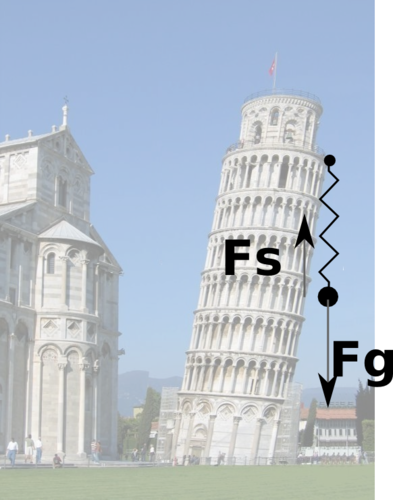# Spring, gravity, damping: source code

```# ...
variables   => {
acceleration  => { \$:gravity + \$:spring + \$:damping },
gravity       => { -9.81 },
spring        => { - 2 * \$:height },
damping       => { - 0.2 * \$:velocity },
},
# ...
```

# Spring, gravity, damping: results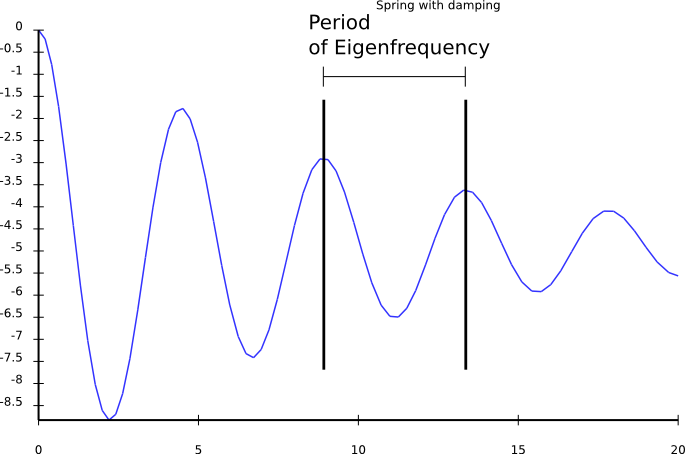# Further extensions

• Let's add an external, driving force
• Example: motor, coupled through a second spring

# External driving force: Code

```sub MAIN(\$freq) {
my \$m = Math::Model.new(
# ...
variables   => {
acceleration  => { \$:gravity + \$:spring
+ \$:damping + \$:ext_force },
gravity       => { -9.81 },
spring        => { - 2 * \$:height },
damping       => { - 0.2 * \$:velocity },
ext_force     => { sin(2 * pi * \$:time * \$freq) },
},
# ...
);

my %h = \$m.integrate(:from(0), :to(70), :min-resolution(5));
\$m.render-svg("spring-freq-\$freq.svg",
:title("Spring with damping, external force at \$freq"));

```

# Driving force: low frequency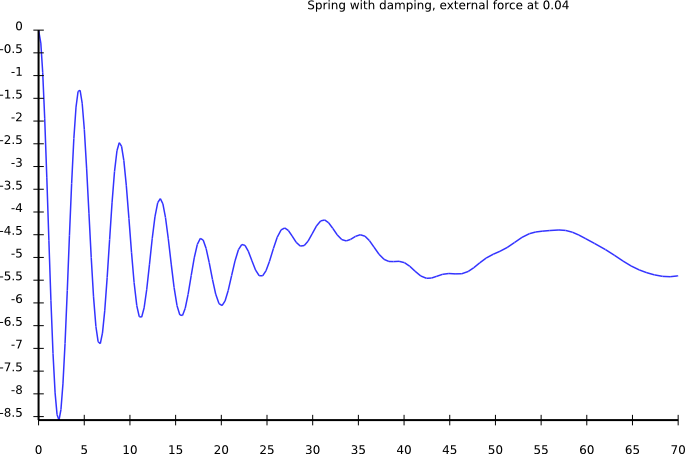# Driving force: higher frequency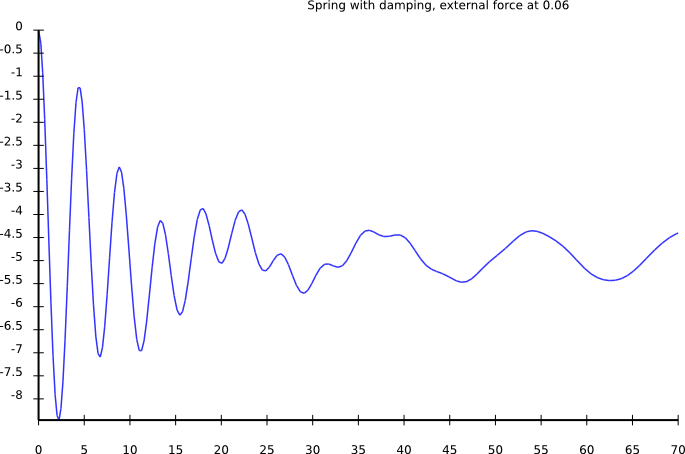# Driving force: higher frequency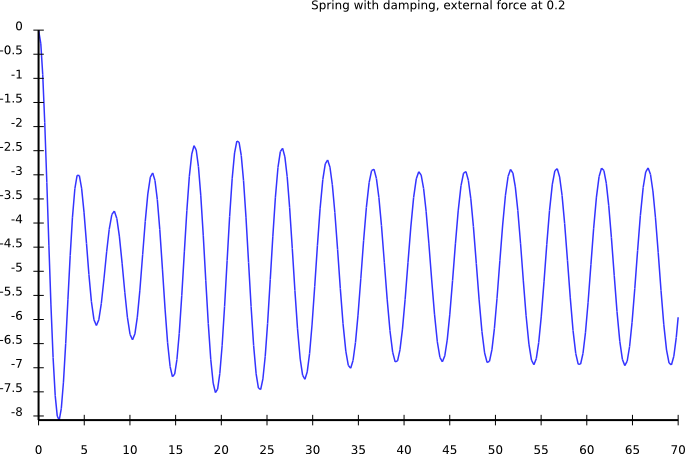# Driving force: higher frequency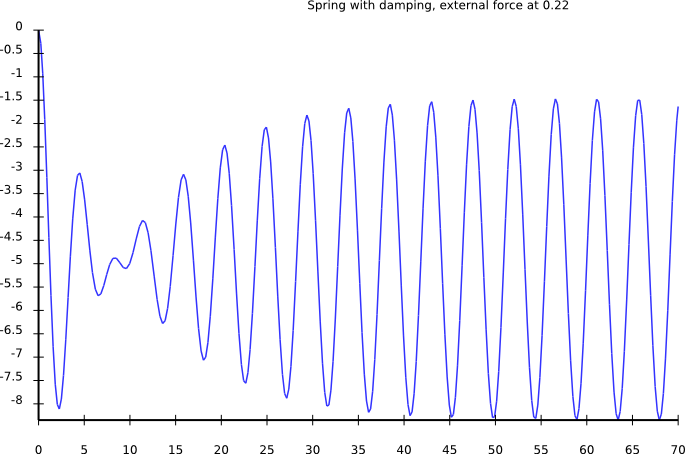# Driving force: higher frequency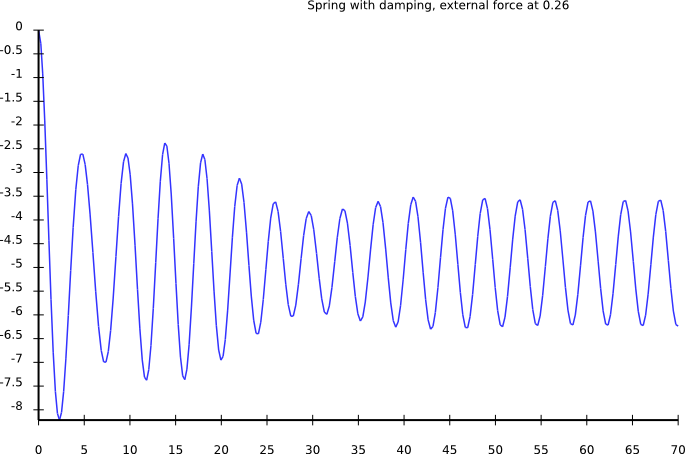# Driving force: higher frequency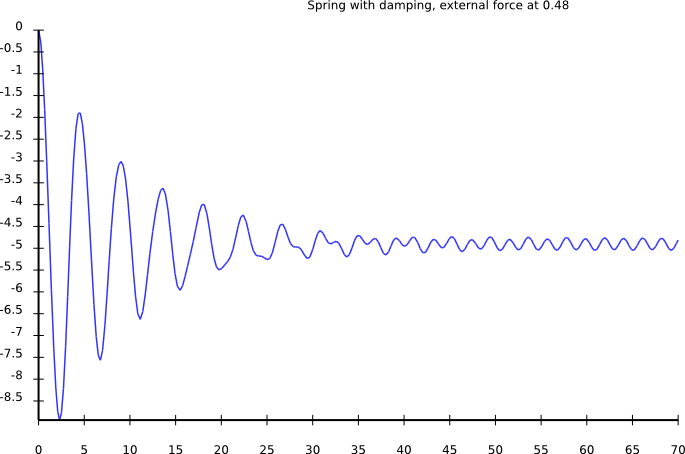# External driving force: Observations

• amplitude low for small frequencies
• amplitude high for driving freq = eigen freq
• amplitude goes to zero for very high frequencies

# Amplitude vs. Frequency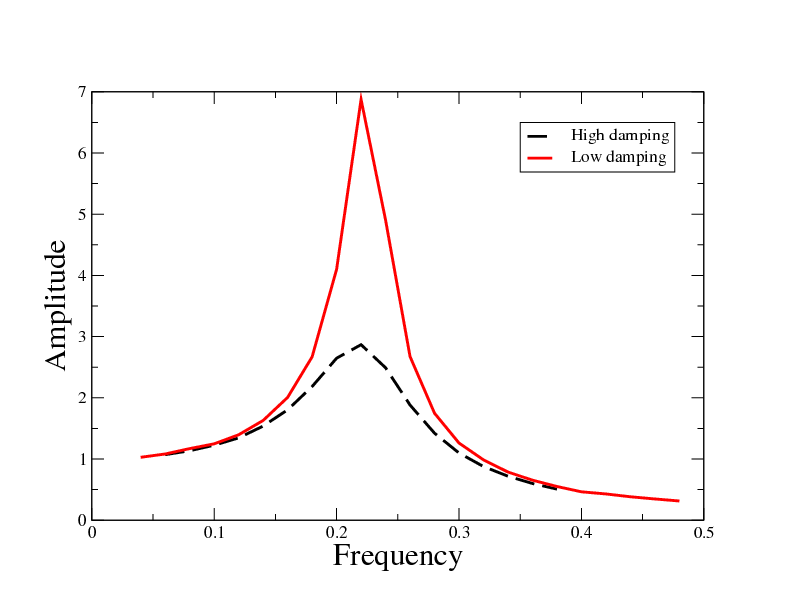# Resonance controls ...

• tune of music instruments
• light absorption, thus color of objects
• heat transport in solids (phonons are lattice resonances)
• everything else :-)

# String theories

• some physicists say that particles are just resonances
• the things that move are called "strings"
• think of it what you want :-)

# Limits of Math::Model

• some fields of physics require other mathematical techniques
• many need partial differential equations
• no quantum mechanics
• no fluid dynamics

# Summary

• physical models: simplifcation to essentials
• `Math::Model` integrates models for you
• oscillator: initial motion + force in opposite direction
• resonance if driving frequency is close to eigen frequency